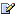CTK Exchange Front Page Movie shortcuts Personal info Awards Reciprocal links Terms of use Privacy Policy Cut The Knot! MSET99 Talk Games & Puzzles Arithmetic/Algebra Geometry Probability Eye Opener Analog Gadgets Inventor's Paradox Did you know?... Proofs Math as Language Things Impossible My Logo Math Poll Other Math sit's Guest book News sit's Recommend this siteCTK Exchange

 Subject: "Geometry" Previous Topic | Next Topic
 ConferencesThe CTK ExchangeHigh schoolTopic #364Printer-friendly copyEmail this topic to a friend Reading Topic #364
dovid31guest
May-12-07, 07:44 AM (EST)

"Geometry"

 Set up an equation of the straight line that goes through the origin and which forms with the lines whose equations are x-y+12=0 and 2x+y+9=0a triangle whose area is 1.5 square units.I graphed the given lines, which intersect at (-7,5). The line I want is y=mx because it goes through the origin and m, from graphing, seems to be negative.I tried using the distance from a point (-7,5) to a line (y=mx) but am at a loss.Thank you.

Subject     Author     Message Date     IDGeometry dovid31May-12-07 TOPRE: Geometry alexb May-13-07 1RE: Geometry SowrabhMay-17-07 2RE: Geometry dovid31May-21-07 6RE: Geometry dovid31May-21-07 7RE: Geometry mpdlcMay-19-07 3RE: Geometry dovid31May-21-07 5RE: Geometry algerya moyeMay-21-07 4

 Conferences | Forums | Topics | Previous Topic | Next Topic
alexb
Charter Member
2012 posts
May-13-07, 08:17 AM (EST)1. "RE: Geometry"
In response to message #0

 >I tried using the distance from a point (-7,5) to a line >(y=mx) but am at a loss. >Distance along would not help. This will serve as an altitude in the triangle in question. To find the area you also need the base, so you have to find the intersections of y = mx with the two given lines. I do not see how the geometry here may simplify the problem. It appears to laborious to me.Sowrabhguest
May-17-07, 10:59 AM (EST)

2. "RE: Geometry"
In response to message #1

 Ans: m=-27/13dovid31guest
May-21-07, 01:43 PM (EST)

6. "RE: Geometry"
In response to message #2

 Incorrect. Also, there are two answers. See.>Ans: m=-27/13dovid31guest
May-21-07, 01:43 PM (EST)

7. "RE: Geometry"
In response to message #1

 Forgive me, but your reply was unhelpful. See another post from someone who also got the correct answer. Thank you all the same. I also figured it out with help, using the determinant method.>>I tried using the distance from a point (-7,5) to a line >>(y=mx) but am at a loss. >>>>Distance along would not help. This will serve as an >altitude in the triangle in question. To find the area you >also need the base, so you have to find the intersections of >y = mx with the two given lines. I do not see how the >geometry here may simplify the problem. It appears to >laborious to me.

mpdlcguest
May-19-07, 12:02 PM (EST)

3. "RE: Geometry"
In response to message #0

 As you probably remember the area S of a triangle of which the three vertices coordinates are given equal to one half of the determinant formed with them as follows:X1 Y1 1X2 Y2 1 = 2*SX3 Y3 1Then you already got the coordinate of one the vertex (-7, 5) . To obtain the other two, remember since the straight line must pass through the origin both vertices must comply with the equation y = mx besides each given equation, where m is the unknownSo by substitution of y = mx in the two original equations you get :X2 = 12/(m-1) and Y2 = 12m/(m-1) for eq. y = x +12And X3 = -9/(m+2) and Y2 = -9m/(m+2) for eq. y = -2x -9Plugging these coordinates in the determinant above and solving itAfter an easy simplification you get a second degree equation in m as follows50 m2 + 71m + 23 = 0 (m2 means the square of m)Solving it you get the two solution m = -1/2 and m= -23/25I let you to have check the answers by graphic and algebra, I already have done itdovid31guest
May-21-07, 01:43 PM (EST)

5. "RE: Geometry"
In response to message #3

 Thank you - I got this answer with the method you suggested.>As you probably remember the area S of a triangle of which >the three vertices coordinates are given equal to one half >of the determinant formed with them as follows: >>X1 Y1 1 >X2 Y2 1 = 2*S >X3 Y3 1 >>>Then you already got the coordinate of one the vertex (-7, >5) . To obtain the other two, remember since the straight >line must pass through the origin both vertices must comply >with the equation y = mx besides each given equation, where >m is the unknown >>So by substitution of y = mx in the two original equations >you get : >>X2 = 12/(m-1) and Y2 = 12m/(m-1) for eq. y = x +12 >>And >>X3 = -9/(m+2) and Y2 = -9m/(m+2) for eq. y = -2x -9 >>>Plugging these coordinates in the determinant above and >solving it >>After an easy simplification you get a second degree >equation in m as follows >>50 m2 + 71m + 23 = 0 (m2 means the square of m) >>Solving it you get the two solution >> m = -1/2 and m= -23/25 >>I let you to have check the answers by graphic and algebra, >I already have done it

algerya moyeguest
May-21-07, 01:25 PM (EST)

4. "RE: Geometry"
In response to message #0

 This is so easy you have x-y+12=0 and 2x+y+9=0 in my math class I did the same way, so you have (-7,5) and y=mx+b you have to plue it in that you already have.thank you

 Conferences | Forums | Topics | Previous Topic | Next Topic
 Select another forum or conference Lobby The CTK Exchange (Conference)   |--Early math (Public)   |--Middle school (Public)   |--High school (Public)   |--College math (Public)   |--This and that (Public)   |--Guest book (Protected)   |--Thoughts and Suggestions (Public) Educational Press (Conference)   |--No Child Left Behind (Public)   |--Math Wars (Public)   |--Mathematics and general education (Public)You may be curious to have a look at the old CTK Exchange archive.